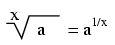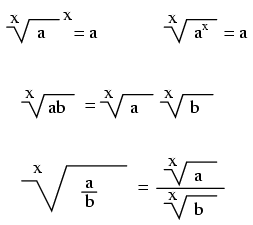# Learning Electronics

Learn to build electronic circuits

# Radicals

### Definition of a radical

When people talk of a "square root," they're referring to a radical with a root of 2. This is mathematically equivalent to a number raised to the power of 1/2. This equivalence is useful to know when using a calculator to determine a strange root. Suppose for example you needed to find the fourth root of a number, but your calculator lacks a "4th root" button or function. If it has a yx function (which any scientific calculator should have), you can find the fourth root by raising that number to the 1/4 power, or x0.25.It is important to remember that when solving for an even root (square root, fourth root, etc.) of any number, there are two valid answers. For example, most people know that the square root of nine is three, but negative three is also a valid answer, since (-3)2 = 9 just as 32 = 9.

### Properties of radicals